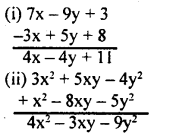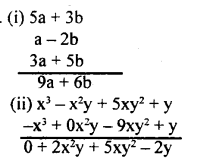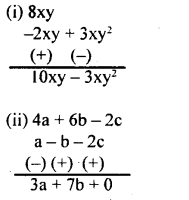# KSEEB Solutions for Class 8 Maths Chapter 2 Algebraic Expressions Ex 2.2

In this chapter, we provide KSEEB SSLC Class 8 Maths Chapter 2 Algebraic Expressions Ex 2.2 for English medium students, Which will very helpful for every student in their exams. Students can download the latest KSEEB SSLC Class 8 Maths Chapter 2 Algebraic Expressions Ex 2.2 pdf, free KSEEB SSLC Class 8 Maths Chapter 2 Algebraic Expressions Ex 2.2 pdf download. Now you will get step by step solution to each question.

## Karnataka Board Class 8 Maths Chapter 2 Algebraic Expressions Ex 2.2

Question 1.
Classify into like terms
4x², (13x) , – 8x3, xy, 6x3, 4y, -74×3, 8xy, 7 xyz, 3x²
Like terms I= (4x², 3x²)
II =$\left(\frac{1}{3} x\right)$
III = -8x3, 6x3, -74×3
IV = (xy, 8xy)
V = (4y)
VI = (7 xyz)

Question 2.
Simplify
i. 7x – 9y + 3 – 3x + 5y + 8
ii. 3x² + 5xy – 4y² + x² – 8xy – 5y²Question 3.
i. 5a + 3b, a – 2b and 3a + 5b
ii. x3 – xy + 5xy² + y , – x3 – 9xy² + y and 3x²y + 9xy²Question 4.
Subtract
i. – 2xy + 3xy² from 8xy
ii. a – b – 2c from 4a + 6a – 2cAll Chapter KSEEB Solutions For Class 8 maths

—————————————————————————–

All Subject KSEEB Solutions For Class 9

*************************************************

I think you got complete solutions for this chapter. If You have any queries regarding this chapter, please comment on the below section our subject teacher will answer you. We tried our best to give complete solutions so you got good marks in your exam.

If these solutions have helped you, you can also share kseebsolutionsfor.com to your friends.

Best of Luck!!